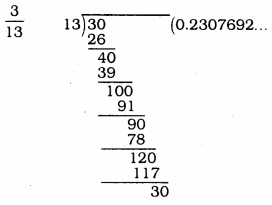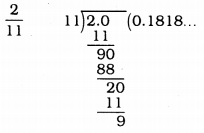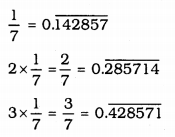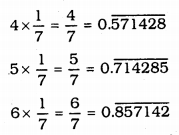# KSEEB Solutions for Class 9 Maths Chapter 1 Number Systems Ex 1.3

KSEEB Solutions for Class 9 Maths Chapter 1 Number Systems Ex 1.3 are part of KSEEB Solutions for Class 9 Maths. Here we have given Karnataka Board Class 9 Maths Chapter 1 Number Systems Exercise 1.3.

## Karnataka Board Class 9 Maths Chapter 1 Number Systems Ex 1.3

Question 1.
Write the following in decimal form and say what kind of decimal expansion each has :(i)Terminating decimal expansion.
(ii)Non-terminating decimal expansion.
\begin{aligned} \therefore \quad \frac{1}{11} &=0.090909 \ldots \\ &=0 . \overline{09} \end{aligned}
(iii)∴ Terminating decimal expansion.
(iv)$$0 . \overline{23069}$$ the bar above the digits indicates the block of digits that repeats.
∴ This is Non-terminating, repeating decimal.
(v)Here, $$0 . \overline{18}$$ is block of digits that repeats.
∴ This is non-terminating, repeating decimal.
(vi)$$\frac{329}{400}=0.8225$$ This is terminating decimal because remainder is zero.

Question 2.
You know that$$\frac{1}{7}=0 . \overline{142857}$$. Can you predict what the decimal expansions of $$\frac{2}{7}, \frac{3}{7}, \frac{4}{7}, \frac{5}{7}, \frac{6}{7}$$ doing the long division ? If so, how? (Hint: Study the remainders while finding the value of $$\frac{1}{7}$$ carefully.).Here the first digit in decimal continued repeated numbers afterward.

Question 3.
Express the following in the form $$\frac{p}{q}$$, where p and q are integers and q ≠ 0. (p, q ϵ Z, q ≠ 0).
(i) $$0 . \overline{6}$$
(ii) $$0 . \overline{47}$$
(iii) $$0 . \overline{001}$$
(i) $$0 . \overline{6}$$ = 0.6666……….
Let x = 0.6666………….
∴ x = 0.6666………..
Multiplying both sides by 10,
10x = 6.666…..
10x = 6 + 0.666
10x = 6 + x
10x – x = 6
9x = 6
$$x=\frac{6}{9}=\text { form of } \frac{\mathrm{p}}{\mathrm{q}}$$
∴ $$0 . \overline{6}=\frac{2}{3}$$

(ii) $$0 . \overline{47}$$ = 0.4777…..
Let x = $$0 . \overline{47}$$ then x = 0.4777……..
Here digit 4 is not repeating but 7 is repeated.
Let x = 0.4777
Multiplying both sides by 10.
10x = 4.3 + x
10x -x = 4.3
9x = 4.3
Multiplying both sides by 10.
90x = 43
∴ $$0.4 \overline{7}=\frac{43}{90}$$

(iii) $$0 . \overline{001}$$ x = 0.001001……….
Multiplying both sides by 1000
1000x = 1.001001
1000x = 1 + x
1000x – x = 1
999x = 1
$$x=\frac{1}{999}$$
∴ $$0 . \overline{001}=\frac{1}{999}$$

Question 4.
Express 0.99999…….. in the form $$\frac{p}{q}$$ , Are you surprised by your answer ? With your teacher and classmates discuss why the answer makes sense.
Express 0.99999 in the form of $$\frac{p}{q}$$,
Let x = 0.99999 …
Multiplying by 10.
10x = 9.9999……
10x = 9 + 0.9999…….
10x = 9 + x
10x – x = 9
9x = 9
$$x=\frac{9}{9}, x=1=\frac{1}{1}$$
∴ (p = 1 & q = 1)
0.99999 …… goes on forever, so there is no gap between 1 and 0.99999 ………… hence they are equal.

Question 5.
What can the maximum number of digits be in the repeating block of digits in the decimal expansion of $$\frac{1}{17}$$ ? Perform the division to check your answer:
Expansion of $$\frac{1}{17}$$ in decimal:$$\therefore \quad \frac{1}{17}=0 . \overline{0588235294117647}$$
Here repeated numbers are 16.

Question 6.
Look at several examples of rational numbers in the form $$\frac{p}{q}$$ (q≠0), where p
and q are integers with no common factors other than 1 and having terminating decimal representations (expansions). Can you guess what property q must satisfy?
Rational numbers in the form $$\frac{p}{q}$$ (q ≠ 0) where P and q are integers with no common factors other than 2 or 5 or both.
OR
Prime factors of q have powers of 2 and prime factors of q have powers of 5 or both.

Question 7.
Write three numbers whose decimal expansions are non-terminating non-recurring.
Three numbers whose decimal expansions are non-terminating, non-recurring are
i) 0.123123312333
ii) 0.20200200020000
iii) 0.56566566656666

Question 8.
Find three different irrational numbers between the rational numbers $$\frac{5}{7}$$ & $$\frac{9}{11}$$ .
$$\frac{5}{7}=0 . \overline{714285} \quad \frac{9}{11}=0 . \overline{81}$$
Three irrational numbers between $$0 . \overline{714285}$$ and $$0 . \overline{81}$$ are :
i) 0.72720720072000………….
ii) 0.7357355735555……………..
iii) 0.760760076000………………

Question 9.
Classify the following numbers as rational or irrational :
(i) $$\sqrt{23}$$
(ii) $$\sqrt{225}$$
(iii) 0.3796
(iv) 7.478478
(v) 1.101001000100001…
i) $$\sqrt{23}$$ = 4.7958………. This is not terminating or non-recurring decimal.
∴ $$\sqrt{23}$$ is an irrational number.
ii) $$\sqrt{23}$$ = 15 this is a rational number.